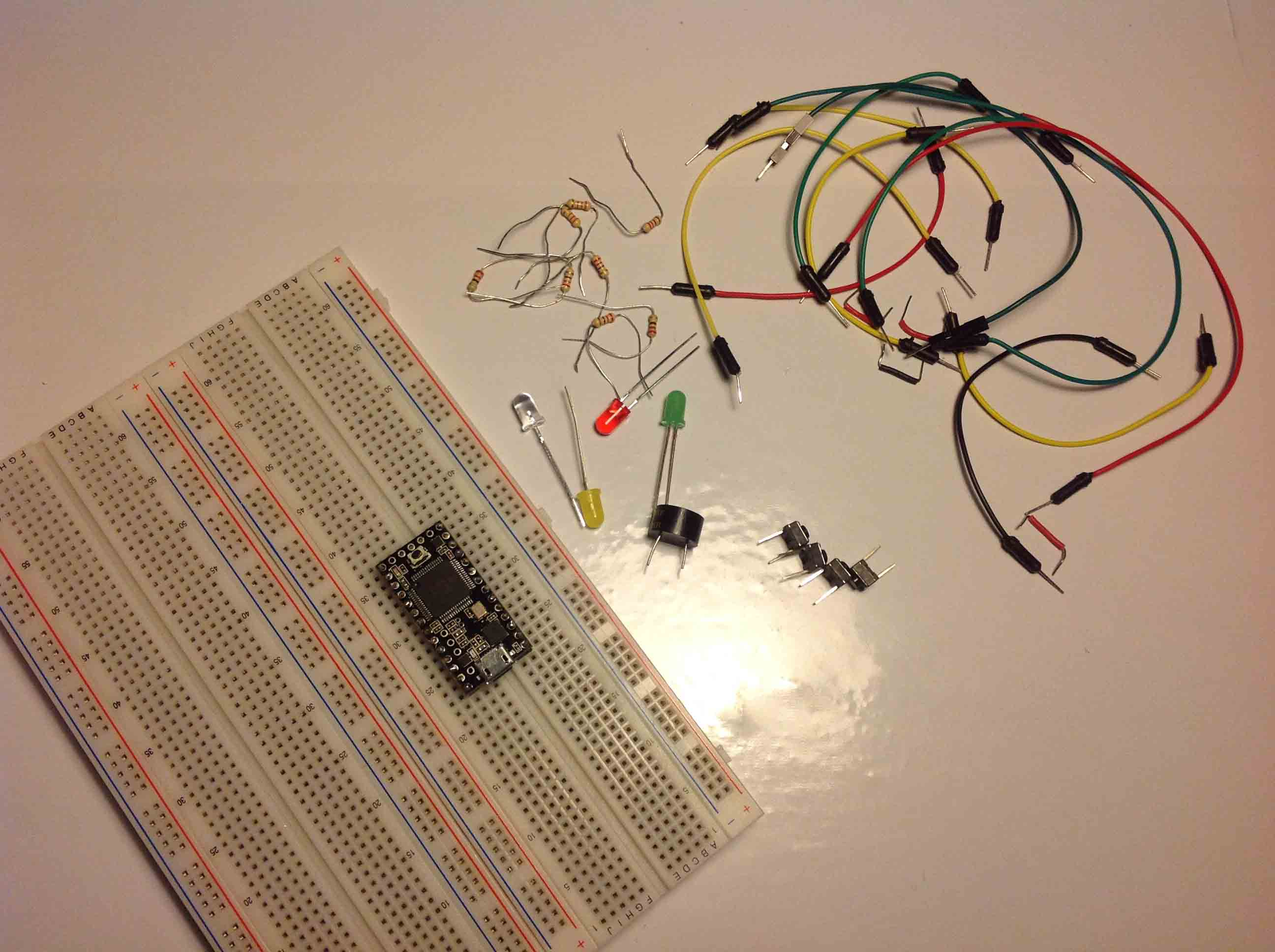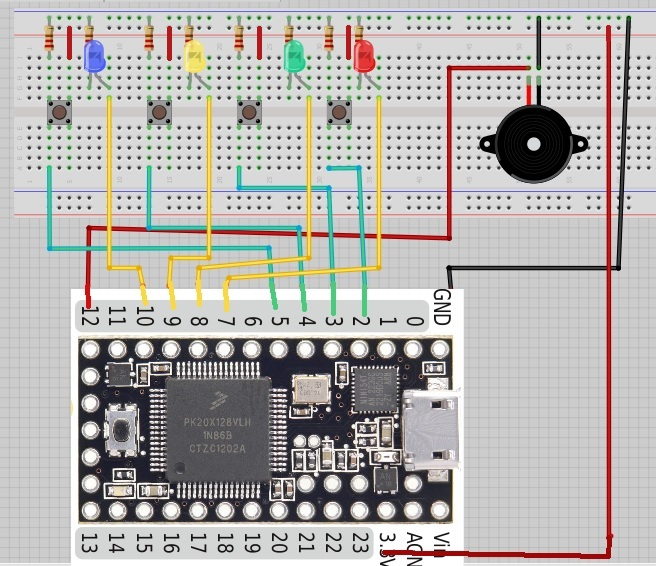# Teensy/Arduino – Memory Game

This project is all about creating a simple game to test your memory.

I’ll be using a Teensy 3.0 board. If you want to know more about this board please click here to read a Getting Started Guide I’ve created a few days ago. This project is also 100% compatible with the Arduino.

## Parts RequiredYou can use the preceding links or go directly to MakerAdvisor.com/tools to find all the parts for your projects at the best price!## Schematics

• Teensy• ArduinoThis code works with Teensy and with the Arduino.

``````/*
Memory Game with Arduino
Based on a project by Jeremy Wilson
Modified by Rui Santos
Visit: https://randomnerdtutorials.com
*/

// Constants
const int button1 = 2;          // 1st button controls Blue LED
const int button2 = 3;          // 2nd button controls Yellow LED
const int button3 = 4;          // 3rd button controls Green LED
const int button4 = 5;          // 4th button controls Red LED
const int led1 = 7;             // Blue LED
const int led2 = 8;             // Yellow LED
const int led3 = 9;             // Green LED
const int led4 = 10;            // Red LED
const int buzzer = 12;          // Buzzer Output
const int tones[] = {1915, 1700, 1519, 1432, 2700}; // tones when you press the LED's - the last one is when you fail.

// Variables
int buttonState[] = {0,0,0,0};         // current state of the button
int lastButtonState[] = {0,0,0,0};     // previous state of the button
int buttonPushCounter[] = {0,0,0,0};

void playTone(int tone, int duration) {
for (long i = 0; i < duration * 1000L; i += tone * 2) {
digitalWrite(buzzer, HIGH);
delayMicroseconds(tone);
digitalWrite(buzzer, LOW);
delayMicroseconds(tone);
}
}

void setup() {
// initialize inputs :
pinMode(button1, INPUT);
pinMode(button2, INPUT);
pinMode(button3, INPUT);
pinMode(button4, INPUT);
// initialize outputs:
pinMode(led1, OUTPUT);
pinMode(led2, OUTPUT);
pinMode(led3, OUTPUT);
pinMode(led4, OUTPUT);
pinMode(buzzer, OUTPUT);
// initialize serial communication for debugging:
//Serial.begin(9600);
}
int game_on = 0;
int wait = 0;
int currentlevel = 1; // This is the level (also the number of button presses to pass to next level)
long rand_num = 0; //initialize long variable for random number from 0-100.
int rando = 0; //initialize random integer for loopgame_on. Will be from 1-4 later.
int butwait = 500; //amount of time to wait for next button input (ghetto de-bounce)
int ledtime = 500; //amount of time each LED flashes for when button is pressed
int n_levels = 10; //number of levels until the game is won
int pinandtone = 0; //This integer is used when the sequence is displayed
int right = 0; //This variable must be 1 in order to go to the next level
int speedfactor = 5; //This is the final speed of the lights and sounds for the last level. This increases as more games are won
int leddelay = 200; //Initializing time for LED. This will decrease as the level increases

void loop() {

int n_array[n_levels];
int u_array[n_levels];

int i;
//clears arrays both "n_array" and "u_array" and starts a new game
if (game_on == 0){
for(i=0; i<n_levels; i=i+1){
n_array[i]=0;
u_array[i]=0;
rand_num = random(1,200);
if (rand_num <= 50)
rando=0;
else if (rand_num>50 && rand_num<=100)
rando=1;
else if (rand_num>100 && rand_num<=150)
rando=2;
else if (rand_num<=200)
rando=3;
//saves a random number in our n_array
n_array[i]=rando;
}
game_on = 1;

}

//shows the user the current sequence
if (wait == 0){
delay (200);
i = 0;
for (i = 0; i < currentlevel; i= i + 1){
leddelay = ledtime/(1+(speedfactor/n_levels)*(currentlevel - 1));
pinandtone = n_array[i];
digitalWrite(pinandtone+7, HIGH);
playTone(tones[pinandtone], leddelay);
digitalWrite(pinandtone+7, LOW);
delay(100/speedfactor);
}
wait = 1;
}
i = 0;
int buttonchange = 0;
int j = 0; // This is the current position in the sequence
while (j < currentlevel){
while (buttonchange == 0){
for (i = 0; i < 4; i = i + 1){
buttonchange = buttonchange + buttonState[i];
}
}
for (i = 0; i < 4; i = i + 1){
if (buttonState[i] == HIGH) {
digitalWrite(i+7, HIGH);
playTone(tones[i], ledtime);
digitalWrite(i+7, LOW);
wait = 0;
u_array[j]=i;
buttonState[i] = LOW;
buttonchange = 0;
}
}
if (u_array[j] == n_array[j]){
j++;
right = 1;
}
else{

right = 0;
i = 4;
j = currentlevel;
wait = 0;
}
}

if (right == 0){
delay(300);
i = 0;
game_on = 0;
currentlevel = 1;
for (i = 0; i < 4; i = i + 1){
digitalWrite(i+7, HIGH);
}
playTone(tones, ledtime);
for (i = 0; i < 4; i = i + 1){
digitalWrite(i+7, LOW);
}
delay (200);
for (i = 0; i < 4; i = i + 1){
digitalWrite(i+7, HIGH);
}
playTone(tones, ledtime);
for (i = 0; i < 4; i = i + 1){
digitalWrite(i+7, LOW);
}

delay(500);
game_on = 0;
}

//if you insert the right sequence it levels up
if (right == 1){
currentlevel++;
wait = 0;
}
//if you finish the game
if (currentlevel == n_levels){
delay(500);
// The following is the victory sound:
int notes[] = {2, 2, 2, 2, 0, 1, 2, 1, 2};
int note = 0;
int tempo[] = {200, 200, 200, 400, 400, 400, 200, 200, 600};
int breaks[] = {100, 100, 100, 200, 200, 200, 300, 100, 200};
for (i = 0; i < 9; i = i + 1){
note = notes[i];
digitalWrite(note+7, HIGH);
playTone(tones[note], tempo[i]);
digitalWrite(note+7, LOW);
delay(breaks[i]);
}
//sets game_on to 0, so it restarts a new game
game_on = 0;
currentlevel = 1;
n_levels = n_levels + 2;
speedfactor = speedfactor + 1;
}

}
``````

View raw code

Did you enjoy this project?

Thanks for reading, you can contact me by leaving a comment. If you like this post probably you might like my next ones, so please support me by subscribing my blog and my Facebook Page.#### [eBook] Build Web Servers with ESP32 and ESP8266 (2nd Edition)

Build Web Server projects with the ESP32 and ESP8266 boards to control outputs and monitor sensors remotely. Learn HTML, CSS, JavaScript and client-server communication protocols DOWNLOAD »

Build Web Server projects with the ESP32 and ESP8266 boards to control outputs and monitor sensors remotely. Learn HTML, CSS, JavaScript and client-server communication protocols DOWNLOAD »

### 8 thoughts on “Teensy/Arduino – Memory Game”

1.Hi there!
I think you missed in the drawing the 3.3V wires from the button switches to 3.3V. Also the 3.3V wire is shorted to the GND line on the breadboard.

Otherwise looks great! Thanks for sharing!

Itzik

•Hi Itzik!
I didn’t notice I made that mistake and forgot some of the wiring, I just updated the schematics now.
I think it’s everything correct.

Thanks for the help.
Have a nice day,
Rui

2.Hi Rui!

Now it works great! Still, need to work on the sounds some more 🙂
Can you please EMail me the schematic in Fritz format? Will help me a lot.

Thank you very much and have a great 2014!
Itzik

3.Some extremely valid info! I appreciate you penning this post plus the rest of
the website is very good.

4.Thanks dude, you did a great job!

•